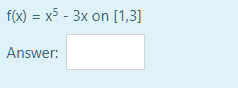Answer should be up to one decimal place only.

f(1)=-2 and f(3)=243-9=234.

We are looking for c where f(c)=0. Since f(1)<0 and f(3)>0, f(c)=0 when c is between 1 and 3, so we take the average of 1 and 3=(1+3)/2=2. f(2)=32-6=26, so f(c)=0 when c is between 1 and 2, that is, 1.5.

f(1.5)=243/32-9/2=3.09375, so f(c)=0 when c is between 1 and 1.5, that is 1.25.

f(1.25)=-0.69824..., so f(c)=0 when c is between 1.25 and 1.5, that is, 2.75/2=1.375.

f(1.375)=0.7899 approx, so f(c)=0 when c is between 1.25 and 1.375, that is, 2.625/2=1.3125. c≃1.3.

f(1.3)=-0.19 which is close to zero.

by Top Rated User (1.1m points)